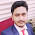## Monday, October 25, 2010

### STATISTICAL ANALYSIS: NONPARAMETRIC TESTS

Advantages and Disadvantages of Nonparametric Tests
The tests of statistical significance discussed in the two previous sections are known as parametric statistics. A parameter, you will recall, is a population score, whereas a statistic is a score for a sample randomly drawn from the population. Parametric statistics make certain assumptions about population parameters. One assumption is that the scores in the population are normally distributed about the mean; another assumption is that the population variances of the comparison groups in one’s study are approximately equal. When large deviations from these assumptions are present in the research data, parametric statistics should not be used. Instead, one of the nonparametric statistics should be selected since, as their name implies, they do not make any assumptions about the shape or variance of population scores.

Parametric statistics assume that the scores being analyzed are derived from a measure that has equal intervals. The student will recall from our discussion in the previous chapter that most continuous scores meet this criterion. When scores are dichotomous or in the form of categories or ranks, one of the nonparametric statistics should be used for data analysis. In the next sections we shall discuss the common types of nonparametric statistics used to analyze data in the form of ranks, frequency counts, or dichotomies.

When research data meet the assumption of being interval scores but do not meet the assumptions of normal distribution and variance homogeneity, it is still advisable to use one of the parametric statistics presented earlier in the chapter. The main reasons for recommending the use of parametric statistics in these situations are that: (1) studies have shown that moderate departure from the theoretical assumptions has very little effect upon the value of the parametric technique; (2) nonparametric statistics are generally less powerful, that is, they require larger samples in order to yield the same level of significance, and (3) for many of the problems encountered in educational research, suitable non-parametric tests are not available.

The Chi-Square Test
Chi-square (x2) is a nonparametric statistical test that is used when the research data are in the form of frequency counts. These frequency counts can be placed into two or more categories. Chi-square was used to investigate one of the objectives of the test-anxiety study by Culler and Holahan. This objective involved replicating previous research findings that high test anxiety is associated with a decrement in CPA.

The researchers formed two categories of CPA: 2.00 or higher, and 1.99 or lower. The reason for splitting GPA,i a continuous variable, into two categories is that “a GPA of 2.00 (On a scale of 0—4) is generally considered a cutoff for success in college, since it is a requirement for graduation.”21 After forming the two categories, the researchers counted the number of high test-anxious and low test-anxious students who fell into each category. The frequency counts are shown in table l3.6. Next, the frequency counts were converted to percentages. For example, 19 high test-anxious students had GPAs lower than 2.00. The total number of high test-anxious students is 65. Nineteen divided by 65 is 29 percent, as shown in table 13.6.

The percentages indicate clearly that high test-anxious students are much dren is a discrete variable (because a family can have 3 or 4 children but not 3½). Appearance before juvenile court is similarly a situation that has either occurred or not occurred and is also discrete. Under these conditions, chi-square is the appropriate test of statistical significance.
Chi-square is equally useful. when the traits or characteristics being considered are actually continuous variables that have been categorized. This was the case with the CPA scores used in the chi-square analysis shown in table 13.6. CPA is a continuous variable because GPAs can take on any value within a given range (in this study, between 0.00 and 4.00). The researchers were justified in transforming CPA into a categorical variable, though, because each category has personal and social significance: failure versus success in college coursework.

Other Nonparametric Tests
Of the nonparametric tests of significance, chi-square is probably the most frequently used by educational researchers in causal-comparative studies. Other non-parametric tests are sometimes used, particularly when the research data are in the form of rant-order scores or interval scores that grossly violate the parametric test assumptions of normality and homogeneity of variance.

The Mann-Whitney U test can be used to determine whether the distributions of scores of two independent samples differ significantly from each other. If U is statistically significant, it means that the “bulk” of scores in one population is higher than the bulk of scores in the other population. The two populations are represented by the two independent samples on which the U test is made.

The Wilcoxon signed-rank test is used to determine whether the distributions of scores in two samples differ significantly from each other when the scores of the samples are correlated (either through matching or because repeated measures are taken on the same sample). The Wilcoxon test is analogous to the t test for correlated means except that it makes no assumptions regarding the shape of the score distribution or homogeneity of variance between the two sets of scores. if more than two groups of subjects are to be compared, a nonparametric one-way analysis of variance (the Kruskal-WaLlis test) can be used.

#### 1 comment:

1.When large deviations from these assumptions are present in the research data, parametric statistics should not be used. Instead, one of the nonparametric statistics should be selected since, as their name implies, they do not make any assumptions about the shape or variance of population scores. fengshui